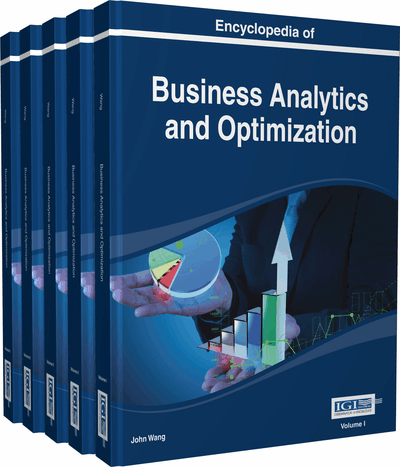# Mathematical Programming Models for Classification

Minghe Sun (College of Business, University of Texas at San Antonio, USA)
DOI: 10.4018/978-1-4666-5202-6.ch136

## Abstract

Mathematical programming models for discriminant and classification analysis are presented. Specifically, linear programming and mixed integer programming approaches are discussed. For each approach, two-class classification models and multi-class classification models are discussed. The emphasis is on the formulations of these mathematical programming models rather than on their performances. Two illustrative examples, one for two-class and the other for multi-class classification, are used to demonstrate the formulations of these mathematical programming models. An example is used to demonstrate the formulation after a mathematical programming model is presented.
Chapter Preview
Top

## Introduction

Discriminant and classification analysis has long been fundamental scientific research and practical applications. Discriminant analysis studies the differences between two or more classes of objects represented by measurements, or variables, of different characteristics or attributes. Classification analysis assigns new observations into the classes based on the measurements of the characteristics. Applications of discriminant and classification analysis are diverse. For example, reported applications in business include financial management, human resource management, and marketing; applications in biology and medicine include patient classification, disease diagnosis and species classification; and applications in environment and geography include remote sensing image pattern classification and pollution control.

Based on known values of the attributes or variables and known class memberships of the observations in a sample, classification or discriminant functions are constructed. The attribute values of an observation can be evaluated by these classification or discriminant functions to obtain discriminant scores based on which the observation is assigned to a class. Statistical techniques, such as Fisher’s linear discriminant function (Fisher, 1936), Smith’s quadratic discriminant function (Smith, 1947) and logistic regression (Hand, 1981), have been standard tools for this purpose. Statistical methods perform well and can provide measures for uncertainty when the data analyzed satisfy the underlying assumptions, such as multivariate normality and equal covariance matrices of the independent variables, although minor deviations from these assumptions do not severely affect the performance of these techniques. More recently, other techniques, such as mathematical programming (MP) (Freed & Glover, 1981a, 1981b; Hand, 1981), neural networks (Stern, 1996) and classification trees (Breiman et al., 1984), have become alternative tools to rival statistical techniques. Support vector machine (SVM) (Vapnik, 1995, 1998), as a machine learning technique, is a revolutionary development in classification analysis. SVMs use quadratic programming techniques. Sun (2014) provides an introduction to some SVM models. A spectrum of techniques is needed because no single technique always outperforms others under all situations (Johnson & Wichern, 1988).

MP approaches attracted interests from researchers because these approaches, as nonparametric methods, do not make strict assumptions about the data analyzed, are less influenced by outlier observations and are flexible in incorporating restrictions. The publication of the original linear programming (LP) models for two-class classification (Freed & Glover, 1981a; Hand, 1981) inspired a series of studies. Some of these studies reported pathologies of the earlier MP models, provided diagnoses, and offered remedies (Markowski & Markowski, 1985; Freed & Glover, 1986; Glover et al., 1988; Cavalier et al., 1989; Glover, 1990; Koehler, 1989a, 1989b, 1990, 1991). The different MP models introduced in the literature include LP, mixed integer programming (MIP), goal programming, nonlinear programming (Erenguc & Koehler, 1990; Stam & Joachimsthaler, 1990; Stam, 1997), multiple objective programming (Stam, 1990; Sun & Xiong, 2003) and quadratic programming (Vapnik, 1995, 1998) models.

## Key Terms in this Chapter

Linear Programming: Mathematical programming models and associated solution techniques where only linear terms of the decision variables are in the objective functions and constraints.

Mixed Integer Programming: Mathematical programming models and associated solution techniques where some of the decision variables are required to be integers in the final solution.

Mathematical Programming: Mathematical models and associated solution techniques used to find one or more optimal solutions within a set of restrictions based on one or more criteria. A typical mathematical programming model has one or more objective functions, a number of decision variables and a set of constraints.

Classification Analysis: Constructing classification or discriminant functions to separate objects in two or more classes using variables measuring the characteristics of the objects and assigning new objects to the classes by evaluating the values of the variables measuring the objects using the constructed classification or discriminant functions.

## Complete Chapter List

Search this Book:
Reset